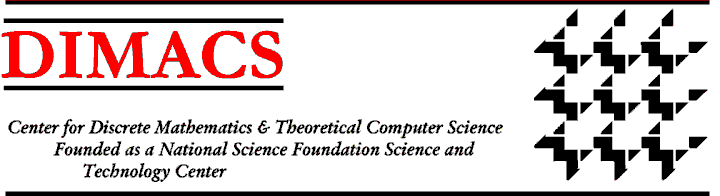### DIMACS/DIMATIA/Rényi Working Group on Algebraic and Geometric Methods in Combinatorics III

#### Third Meeting: November 7 - 9, 2005 Location: DIMACS Center, CoRE Building, Rutgers University

Organizers:
Jaroslav Nesetril, Charles University, nesetril at kam.mff.cuni.cz
Fred Roberts, DIMACS, froberts@dimacs.rutgers.edu

The third meeting of the Working Group on Algebraic and Geometric Methods in Combinatorics will include the following topics (among others):

* Algebraic methods
*the study of homomorphisms of graphs, with special emphasis on problems arising from statistical physics
* long range order for graph homomorphisms
* random H-colorings
* phase transitions

* Problems of combinatorial geometry
* finite geometries - blocking sets - defining sets
* geometric graphs - Turan-type problems - generalizations of the Ham Sandwich Theorem
* crossing number of graphs

* Application of algebraic geometry
* ideals of infinite point sets
* nonvanishing theorems for combinatorial applications
* geometric construction of norm graphs
* intersections of diagonal hypersurfaces and algebraic varieties
* explicit constructions of extremal objects
* arrangements of algebraic surfaces
* lower bound proofs
* robust computations,
* hidden convexity
* combinatorial characterizations of algebraic sets
* Szemeridi's LemmaNext: Call for ParticipationWorkshop IndexDIMACS Homepage
Contacting the Center